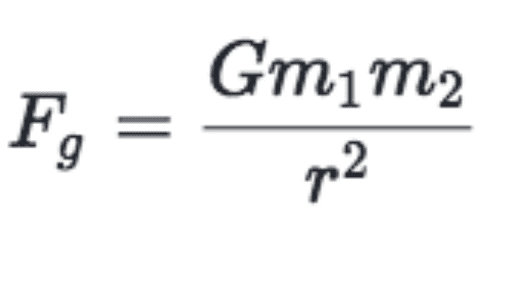# Underwater

Rafael Aiguzhinov
I wonder, what object can be drown to the deepest water ? I mean, the object where gravity is a lot more bigger than force of Archimedes

Mentor
Welcome to PhysicsForums.I wonder, what object can be drown to the deepest water ? I mean, the object where gravity is a lot more bigger than force of Archimedes
Unfortunately your question didn't translate very well. Are you asking about how a sinking object is affected by stronger gravity as it sinks deeper? If so, I don't think the gravitational force increases with depth, since the gravitational attraction of the mass of the water above the sinking object decreases the net downward force on the object.

Is that what you were asking about, or is your question different? Can you give some examples of what you are thinking about? And is the sinking object compressible or not compressible?

•russ_watters
JT Smith
I wonder, what object can be drown to the deepest water ? I mean, the object where gravity is a lot more bigger than force of Archimedes

Anything denser than the densest water in your imagination. If it's the ocean that's not particularly dense and lots of things -- LOTS OF THINGS -- will sink to the bottom.

Being that this is your very first post be prepared for it to sink to the bottom. :-)

Rafael Aiguzhinov
Yes, I meant this

Mentor
Yes, I meant this

Homework Helper
Unfortunately your question didn't translate very well. Are you asking about how a sinking object is affected by stronger gravity as it sinks deeper? If so, I don't think the gravitational force increases with depth, since the gravitational attraction of the mass of the water above the sinking object decreases the net downward force on the object.
If the density of the Earth were uniform and the same as water then the acceleration of gravity would indeed decrease linearly with depth. As you point out, this follows from Newton's spherical shell theorem: The mass of water above the object's depth "does not count" toward the attraction.

Wiki has a nice graph showing how local gravity would scale with depth on:

a. An Earth whose density is uniform.
b. An Earth whose density increases linearly with depth.
c. A model Earth that we believe most closely matches our own.

As one can see from the graph, the real Earth's gravity gets stronger with increasing depth under the ocean's surface. This goes counter to one's untutored expectation.

Both weight and buoyancy ("the force of Archimedes") scale with the local acceleration of gravity. If all other things remain constant, an object that sinks in water at the surface of the ocean where gravity is normal will continue to sink in water at the bottom of the ocean where gravity is slightly higher than that.

Of course, all other things do not remain constant. There are variations in temperature and salinity. There are also pressure effects. Due to increased pressure at great depths, the density of water increases at those depths. This increases buoyancy. But the sinking object is exposed to this same increased pressure. This compresses the object, reducing the buoyant force on the object. Which effect "wins" depends on the compressibility of the object compared to the compressibility of water.

A lump of iron will sink in the deepest water. It will also sink in molten rock. That is essentially the reason why the Earth has an iron core.

Last edited:
••Limebat, Delta2 and berkeman
Limebat
I wonder, what object can be drown to the deepest water ? I mean, the object where gravity is a lot more bigger than force of Archimedes

Ah, maybe my intuition is a tad off, but gravity in simplified classical physics is:Assuming we're going as deep sea as possible (Mariana Trench is about ~7 miles) and the Earth is ~3950 miles, the two masses (mass one at sea level and mass two at ~7 miles lowered) are going to have roughly similar accelerations due to gravity.

For reference, the space station at ~422km apogee, experiences ~90 percent of Earth's gravity. So the roughly ~7 mile difference wouldn't really affect much.

However, this formula is a gross oversimplification of gravity. It doesn't account for the stipulations the post above made^. I would highly encourage further study of the stuff the dude above me posted!

A question similar to yours:

https://physics.stackexchange.com/questions/132935/gravity-beneath-sea-level

*I would recommend you look at the last post on bottom of site to see an elaboration on the shell theorem.

On that note, maybe you're thinking deep sea pressures? I mean, it sounds like you are wondering why extreme forces are acting on an object below sea.

If that's the case:

"Your figure for being below water is not correct. As you descend in the ocean the ambient pressure increases by about 1 atm/10 meters. In a uniform sphere, the gravitational field is linear in the radius, zero at the center. Making the incorrect assumption that the Earth is a uniform sphere, being down 1 km would decrease the gravitational acceleration by about 1637116371, not much change."